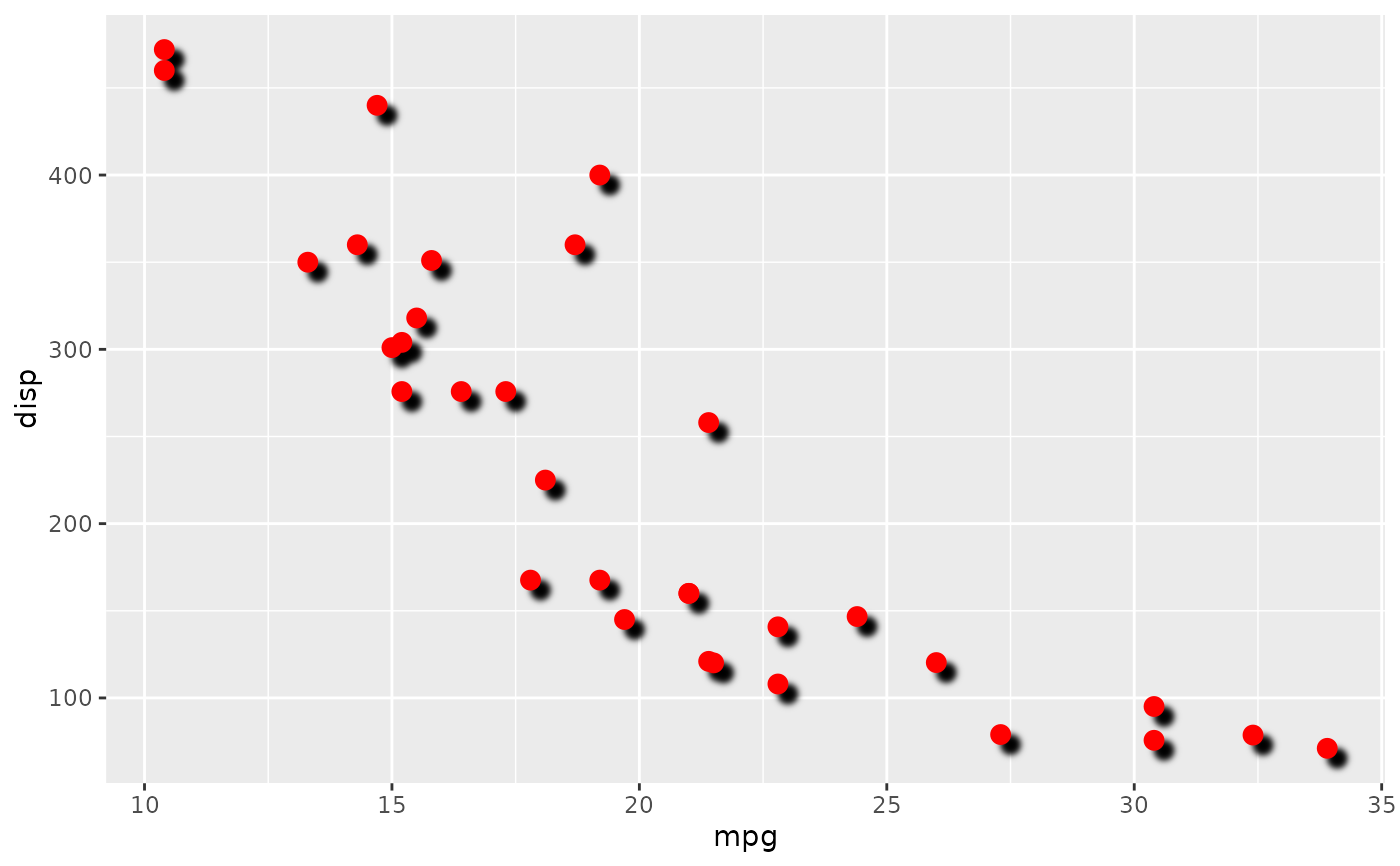This filter applies the familiar drop-shadow effect on elements in a layer. It takes the outline of each shape, offsets it from its origin and applies a blur to it.

with_shadow(
x,
colour = "black",
x_offset = 10,
y_offset = 10,
sigma = 1,
stack = TRUE,
...
)

## Arguments

x

A ggplot2 layer object, a ggplot, a grob, or a character string naming a filter

colour

x_offset, y_offset

The offset of the shadow from the origin as numerics

sigma

The standard deviation of the gaussian kernel. Increase it to apply more blurring. If a numeric it will be interpreted as given in pixels. If a unit object it will automatically be converted to pixels at rendering time

stack

Should the original layer be placed on top?

...

Arguments to be passed on to methods. See the documentation of supported object for a description of object specific arguments.

## Value

Depending on the input, either a grob, Layer, list of Layers, guide, or element object. Assume the output can be used in the same context as the input.

## Examples

library(ggplot2)
ggplot(mtcars, aes(mpg, disp)) +
with_shadow(geom_point(colour = 'red', size = 3), sigma = 3)